## Hydrogen Atom in a Weak Magnetic Field

One way to break the spherical symmetry is to apply an external B field. Lets assume that the field is weak enough that the energy shifts due to it are smaller than the fine structure corrections. Our Hamiltonian can now be written as, whereis the normal Hydrogen problem,is the fine structure correction, andis the term due to the weak magnetic field.

We now run into a problem becausepicks eigenstates of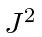andwhile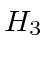picks eigenstates ofand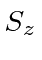. In the weak field limit, we can do perturbation theory using the states of definite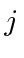. A direct calculation of the Anomalous Zeeman Effect gives the energy shifts in a weak B field.This is the correction, due to a weak magnetic field, which we should add to the fine structure energies.Thus, in a weak field, the the degeneracy is completely broken for the states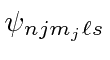. All the states can be detected spectroscopically.The factor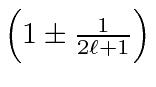is known as the LandeFactorbecause the state splits as if it had this gyromagnetic ratio. We know that it is in fact a combination of the orbital and spin g factors in a state of definite.

In the strong field limit we could use states of definiteandand calculate the effects of the fine structure,, as a correction. We will not calculate this here. If the field is very strong, we can neglect the fine structure entirely. Then the calculation is easy.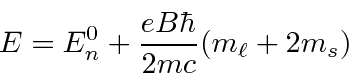In this limit, the field has partially removed the degeneracy inand, but not. For example, the energies of all thesestates are the same.Jim Branson 2013-04-22